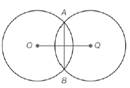Chapter 6.1, Problem 18EElementary Geometry For College St...

7th Edition
Alexander + 2 others
ISBN: 9781337614085

Solutions

Chapter
SectionElementary Geometry For College St...

7th Edition
Alexander + 2 others
ISBN: 9781337614085
Textbook Problem

A B ¯ is the common chord of ⊙ O and ⊙ Q . If A B = 12 and each circle has a radius of length 10 , how long is O Q ¯ ?Exercises 18, 19

To determine

To calculate:

The length of OQ¯.

Explanation

Given:

AB¯ is the common chord of O and Q. If AB=12 and each circle has a radius of length 10.

Theorem used:

1. A radius that is perpendicular to the chord bisects the chord.

2. An angle inscribed in a semicircle is a right angle.

Calculation:

In the circle O, OA¯ and OB¯ are radii and in circle Q, QA¯ and QB¯ are radii. By joining these radii we get a rhombus with sides of length 10.

Thus AQBO is a rhombus with diagonals OQ¯ and AB¯.

We know that the diagonals of a rhombus are perpendicular to each other.

Hence OQ¯ is perpendicular to AB¯.

By the theorem, “A radius that is perpendicular to the chord bisects the chord”, we get OQ¯ bisects AB¯.

Consider, ΔOAQ.

Since AB¯ is bisected by OQ¯ and we have AB=12.

AE=6

Now consider two triangles ΔOAE and ΔQAE.

Here, OA¯QA¯, EA¯EA¯ and AEOAEQ

ΔOAEΔQAE

Since, the two triangles are congruent, we get OE¯QE¯

Still sussing out bartleby?

Check out a sample textbook solution.

See a sample solution

The Solution to Your Study Problems

Bartleby provides explanations to thousands of textbook problems written by our experts, many with advanced degrees!

Get Started

Find more solutions based on key concepts